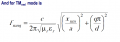The best dimensions for a cylindrical resonant cavity working at 4GHz

Cpar

Joined Dec 20, 2019
3
In this semester I have to design and make a cylindrical resonant cavity working at 4GHz to determine the dielectric constant of a small ball which would be positioned into the cavity. This resonant will be connected to a network analyzer. What dimension should I design and which modes should I use?

Papabravo

Joined Feb 24, 2006
17,616
In this semester I have to design and make a cylindrical resonant cavity working at 4GHz to determine the dielectric constant of a small ball which would be positioned into the cavity. This resonant will be connected to a network analyzer. What dimension should I design and which modes should I use?
So what is the wavelength of a 4 GHz. continuous wave carrier? That would be a good number to know. Is the number you are looking for some number of, or fractions of a wavelength?

Cpar

Joined Dec 20, 2019
3
My problem is that I have to choose a mode to base my calculations - I think of TM010 - so I can determine the exact radius and length of the resonator based on this equation.I have to choose the appropriate mode so I will have big quality factor (Q). Also, at the 2 bases of the cylidrical resonator I have 2 probes for transmition and reception respectively.

Papabravo

Joined Feb 24, 2006
17,616
If you have only 1 equation, but two unknowns, then there are an infinite number of solutions that satisfy the condition. You need something else to constrain the problem and yield a unique solution.

Cpar

Joined Dec 20, 2019
3
I don't have only one equation. This is the one from which I will find the right dimensions, for a specific mode. I 'd appreciate some advice for what (TM or TE) mode to use.

Papabravo

Joined Feb 24, 2006
17,616
I don't have only one equation. This is the one from which I will find the right dimensions, for a specific mode. I 'd appreciate some advice for what (TM or TE) mode to use.
You only showed us one equation. Why are you holding out on us?
Are there any constraints you know of that would favor one mode over another, or is the choice strictly random.